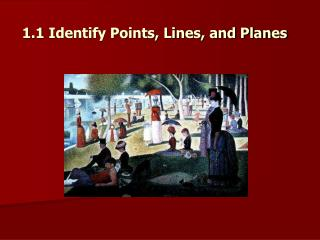DownloadDownload Presentation1.1 Identify Points , Lines, and Planes

# 1.1 Identify Points , Lines, and Planes

Télécharger la présentation## 1.1 Identify Points , Lines, and Planes

- - - - - - - - - - - - - - - - - - - - - - - - - - - E N D - - - - - - - - - - - - - - - - - - - - - - - - - - -
##### Presentation Transcript

1. 1.1 Identify Points, Lines, and Planes

2. Objectives: • Understand and use the basic undefined terms and defined terms of geometry. • Sketch the intersections of lines and planes.

3. Undefined Terms A definitionuses known words to describe a new word. In geometry, some words such as point, line, and plane are undefinedterms. In other words, there is no formal definition for these words, but instead they are explained by using examples and descriptions which allows us to define other geometric terms and properties.

4. 1.1 Points, Lines, & Planes Date Objectives: Notes: Assignment: • undefined terms of geometry • intersections of lines & planes • Point, line and plane are undefined terms Example of what your note page should look like now

5. A point is simply a location. It has no dimension (shape or size), is usually represented by a small dot, and named by a capital letter. Point A

6. A line is a set of points and extends in one dimension. It has no thickness or width, is usually represented by a straight line with two arrowheads to indicate that it extends without end in both directions, and is named by two points on the line or a lowercase script letter. Line A l B AB or line l

7. A planeis a flat surface made up of points. It extends in two dimensions, is usually represented by a shape that looks like a tabletop or wall, and is named by a capital script letter or 3 non-collinear points. You must imagine that the plane extends without end, even though the drawing of a plane appears to have edges. A M C B Plane Plane ABCor plane M

8. Space • Space is a boundless, three dimensional set of all points. It can contain points, lines, and planes.

9. A few more basic concepts using these undefined terms . . . • Collinear points are points that lie on the same line. • Coplanar points are points that lie on the same plane.

10. Name three points that are collinear Solution: D, E and F lie on the same line, so they are collinear. Example 1: H G E F D

11. Name four points that are coplanar. Solution: D, E, F, and G lie on the same plane, so they are coplanar. Also D, E, F, and H are coplanar; although, the plane containing them is not drawn. Example 2: H G E F D

12. Name three points that are not collinear. Solution: There are many correct answers. For instance, points H, E, and G do not lie on the same line. Example 3: H G E F D

13. Another undefined concept in geometry is the idea that there is always a point on a line between two other points on the line. Consider the line AB (symbolized by AB). More . . . A C B

14. A line segment or segment AB (or BA) consists of the endpoints A and B, and all points on AB that are between A and B. Line segments have exact measures but can only be as precise as the smallest unit of the measuring device. Segments CD D C B A AB

15. Rays • To create angles we must first define a ray. A ray is part of a line which has one endpoint and extends infinitely in one direction.

16. Labeling Rays • Rays are named stating the endpoint first and then any other point on the ray. • We could label these rays as AB, AC, or AD but not CA. D C B A

17. More about Rays • If you choose a point on a line, that point determines exactly two rays called opposite rays. Opposite rays share one common point, where they both start. P Q R QP and QR are opposite rays.

18. Intersections of Lines & Planes • Two or more lines intersect if they have a common point. Two or more planes intersect if they have a common line. The intersection of any figures is the set of points the figures have in common.

19. How to sketch a line that intersects a plane in one point Draw a plane and a line. Emphasize the point where they meet. Use dashes to indicate where the line is hidden by the plane. Example 4:

20. How to sketch two planes that intersect in a line. Draw two planes. Emphasize the line where they meet. Use dashes to indicate where one plane is hidden by the other plane. Example 5:

21. Assignment: • Geometry Pg. 5 – 8 #1 – 22, 26 - 32